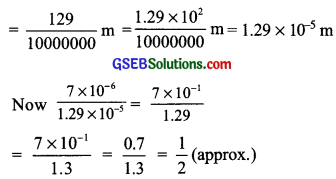# GSEB Solutions Class 8 Maths Chapter 12 Exponents and Powers Intext Questions

Gujarat Board GSEB Textbook Solutions Class 8 Maths Chapter 12 Exponents and Powers Intext Questions and Answers.

## Gujarat Board Textbook Solutions Class 8 Maths Chapter 12 Exponents and Powers

Try These (Page 194)

Question 1.
Find the multiplicative inverse of the following?

1. 2-4
2. 10-5
3. 7-2
4. 5-3
5. 100-100

Solution:

1. The multiplicative inverse of 2-4 is 24 ponents.
2. The multiplicative inverse of 10-5 is 105
3. The multipicative inverse of 7-2 is 72
4. The multipicative inverse of 5-3 is 52
5. The multipicative inverse of 10-100 is 10100Try These (Page 194)

Question 1.
Expand the following number using exponents?
(i) 1025.63
(ii) 1256.249
Solution:Try These (Page 195)

Question 1.
Simpfy and write in exponential form?

1. (-2)-3 × (-2)-4
2. p3 × p-10
3. 32 × 3-5 × 36

Solution:
1. (-2)3 × (-2)-4 = (-2)(-3)+(-4) [∵am × an]
= (-2)-7 or $$\frac{1}{(-2)^{7}}$$

2. p3 × p-10 = (p)3+(-10) = (p)-7 or $$\frac{1}{(10)^{7}}$$

3. 32 × 3-5 × 36 = 32+(-5)+6 = 38-5 = 33

Law II: $$\frac{a^{m}}{a^{n}}$$ = am-n
Example: 5-1 + 5-2 = 5-1-(-2) = 5-1+2 = 51 or 5

Law III: (am)n = amn
Example: (9-1)-3 = 9(-1)×(-3) = 93

Law IV: am × bm = (ab)m
Example: 2-4 × 3-4 = (2 × 3)-4 = 6-4 or $$\frac{1}{6^{4}}$$

Law V: $$\frac{a^{m}}{b^{m}}=\left(\frac{a}{b}\right)^{m}$$
Example: $$\frac{3^{-5}}{7^{-5}}$$ = $$\frac{3}{7}$$-5 or $$\frac{7}{3}$$5

Law VI: a0 = 1
Example:
(i) (-38)0 = 1
(ii) (32456)0 = 1

Try These (Page 199)

Question 1.
Write the following numbers in standard form?

1. 0.000000564
2. 0.0000021
3. 15240000

Solution:
1. 0.000000564 = $$\frac{564}{1000000000}$$
= $$\frac{5.64}{10^{9}} \times 10^{2}$$
= $$\frac{5.64}{10^{7}}$$
= 5.64 × 10-7

2. 0.0000021 = $$\frac{21}{10000000}$$
= $$\frac{2.1 \times 10}{10000000}=\frac{2.1}{1000000}$$
= 2.1 × 10-6
∴ 0.0000021 = 2.1 × 10-6

3. 15240000 = 1524 × 1000
= 1.524 × 1000 × 1000
= 1.524 × 103 × 104
= 1.524 × 107
∴ 15240000 = 1.524 × 107Question 2.
Write all the facts given in the standard form?
Solution:
A number is said to be in the standard form
when it is written as k × 10n,
where 1 ≤ k < 10 and ‘n’ is an integer
A number expressed as the product of a number between 1 and 10 and an integral power of 10.
Example: Compare the size of a red blood cell which is 0.000007 m to that of a plant cell which is 0.0000129 m.
Solution:
Size of red blood cell = 0.000007 m
= $$\frac{7}{1000000}$$
= 7 × 10-6 m
Size of the plant cell = 0.0000129 mThus, the size of a red blood cell is half of the plant cell size.